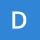Home
IT Knowledge
Inspiration
Languages
EN

# Math - why sin(0) is equal 0?

11 points
Created by:Dollie-Rutledge
476

Explaination why `sin(0)=0` requires to refer to the function definition.

`sin(α)` has been defined as:

Lets suppose that: alpha angle goes to zero (`α -> 0`) and adjacent side is constant (`b = const`).

It causes: opposite side goes to zero too (`a -> 0`) and hypotenuse side goes to adjacent side (`h -> b`). When angle is equal to `0` and `a` is equal to `0` then `0` divided by `h` is equal to `0`. It means `sin(0)` is equal to `0` acording to above definition.

Simple visualisation is presented on below picture: